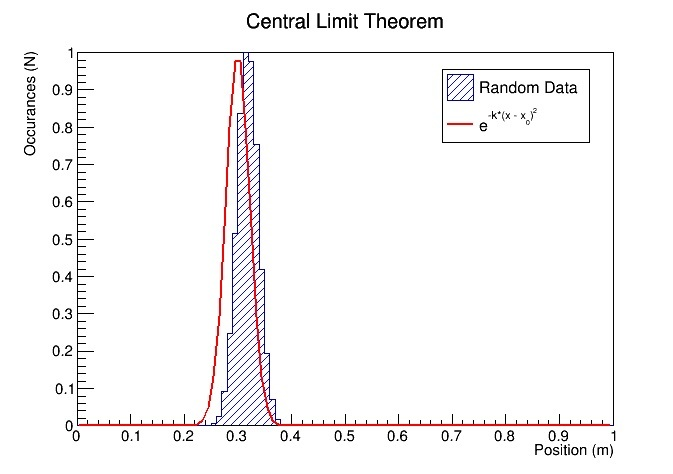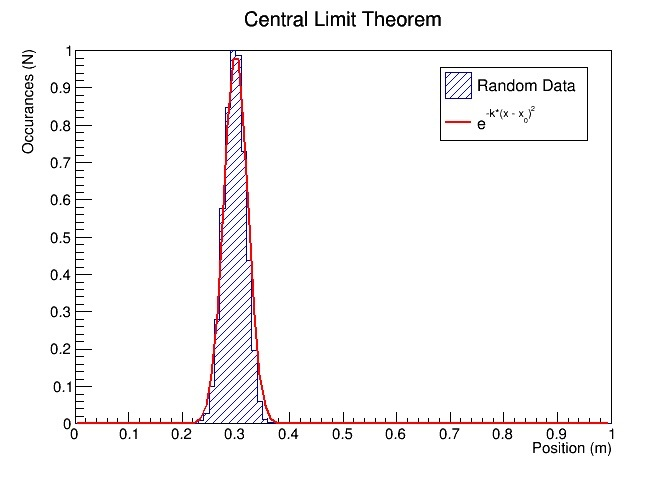# Shift Histogram

I have a histogram that is the distribution of random numbers, which is a gaussian distribution. But I need to fit it to an equation. So I’ve used the standard deviation to change the distribution but now I need to shift the center of the distribution from the normal (.5) to where it should be for my particular function (.3).

f(x) = exp (-1000*(x - 0.3)^2)

I need the center of the distribution to be at 0.3. I would normally do sigma * x + mu, but that doesn’t seem to be working.

In theory I would like to code the change. mu is 0.3 (x0 in my code). And I can use GetMaximumBin to find the center of the distribution and then I would like to shift it from the location determined by GetMaximumBin to 0.3.

I thought that since the center of the distribution with sigma = 1 was .5 and my center with sigma = 0.01977 shoudl be .3 that I should shift it by .2 but that doesn’t quite work. Is there a method to change the center of a distribution in root?

Do I rebin it? I’m basically being forced to teach myself root and I think maybe I’m just not sure what the proper terms are for what I want to do. ANy help would be appreciated.Just through trial and error I’ve determined that this works:

``h->Fill(sigma*number + .180);``

But I’d like to figure out where that number comes from and calculate it so that if something changes I don’t need to go through the trial and error process to find the appropriate value.

To confirm I used GetMaximum to find out that the max bin was located at .12 so the difference between where it was (.12) and where I wanted it (.3) was .180 so my trial and error is back up by the math.

But I still want to calculate it inside my code in case I wanted to change mu.